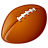# Merging Rasters

485
5
09-20-2013 04:00 AMNew Contributor
I am trying to join two rasters together that have different values so that they are a single dataset with the different values. I have tried using the Mosaic to New Raster tool but this seems to either miss some areas or merge the attributes. Ideally what I want to display is where the two rasters are the same, then parts which are not as 3 different attributes but not removing any of the original data. I have tried using the Equal to but this removes some of the data that I want to display.

I have attached an example of the sort of thing I am trying to produce but this is with 3 different layers. How can I combine all this information and keep the separate attributes and all the data? The blue represents where the two rasters are equal, then the red and the green are where the two rasters are different.

Regards,
Andrew
Tags (3)
5 RepliesbyMVP Regular Contributor
What are the 3 values you want?
val1 = (raster1 = raster2)
val2 = (raster1<>raster2)
which raster value do you retain here?

What is the val3?
Regards,
JimNew Contributor
Both rasters are logical datasets so the three values I am trying to extract are where both rasters = 1, where the first raster = 1 whilst the other raster = 0, then the reverse of this (so when the second raster = 1 but the first raster = 0).

Ideally I want a raster that shows all the data. The rasters have the same extent but do not overlap perfectly so currently I have only been able to produce a raster the shape of the first raster, whilst some of the pixels of the second raster are deleted as they are not contained in the first raster.

ThanksbyMVP Regular Contributor
Con(raster1 = raster2, 0, (raster1 - raster2))
so, output is "0" where raster1 equals raster2, and raster1 - raster 2 where they are not equal.
If raster1 =1 and raster2 = 0, you get 1 as output
If raster1 = 0 and raster2 =1, you get -1 as output
So final output has 0 where the inputs are the same, 1 where raster1 is true and raster2 is false, and -1 where raster1 is false and raster2 is true.
As for extents, select the "Environments" button at the bottom of the tool window, select "Processing Extent" and choose Union of Inputs, to get the combined extent of all the input data.
Regards,
JimNew Contributor
Not sure if it is something I am doing but I don't seem to be able to call the Con statement that you stated using the Con Tool. I am only able to call the attributes in the Input Conditional Raster. Are you suggesting to use the Con tool or should I use something else for this?

Regards,
AndrewbyMVP Regular Contributor
I just use the Map Algebra >> Raster Calculator tool, and type my Con statement in there.